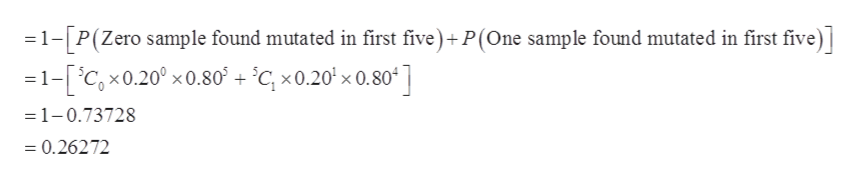# Samples of rejuvenated mitochondria are mutated (defective) with probability 0.2. Find the probability you need to examine at least 6 samples to find 2 samples containing mutations. Report answer to 3 decimal places.

Question
276 views

Samples of rejuvenated mitochondria are mutated (defective) with probability 0.2. Find the probability you need to examine at least 6 samples to find 2 samples containing mutations. Report answer to 3 decimal places.

check_circle

Step 1

In this question, we need to find the probability that at least 6 samples need to examine to find the 2 samples mutated. The probability that a sample is mutated is 0.2.

Step 2

If we need to find the probability that at least 6 samples need to examine to find the 2 samples mutated which means in first 5 samples that have examined only one or...help_outlineImage Transcriptionclose1-P(Zero sample found mutated in first five)+P(One sample found mutated in first five) =1-[iC,x0.20° x0.80 + °Cc, x0.20' x 0.80 ] =1-0.73728 =0.26272 fullscreen

### Want to see the full answer?

See Solution

#### Want to see this answer and more?

Solutions are written by subject experts who are available 24/7. Questions are typically answered within 1 hour.*

See Solution
*Response times may vary by subject and question.
Tagged in

### Other